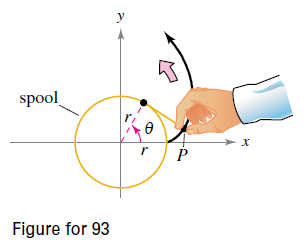Chapter 10.3, Problem 93E

Chapter
Section
Textbook Problem

Involute of a Circle The involute of a circle is described by the endpoint P of a string that is held taut as it is unwound from a spool that does not turn (see figure). Show that a parametric representation of the involute is x = r ( cos θ + θ sin θ )     and   y = r ( sin θ − θ cos θ )To determine

To Prove: Show that the parametric representation of involute of a circle of radius r is

x=r(cosθ+θsinθ) and y=r(sinθθcosθ)

Explanation

Given: The involute of circle is described by the endpoint of string that is held taut as it is unwound from the spool that does not turn (see the below figure)

Formula Used:

Length of the arc of a polar curve =radius×angle

Sum of the three interior angles of a triangle =180

Proof:

Curve traced by point P at the end of string is called the involute of circle.

Draw a perpendicular SA from point S on the x-axis as shown in the figure below.

0AS=90°

Also, draw a perpendicular PV from the point p to the line SA

PVS=90°

Observe that, the length of the segment SP is the amount of string unwounded. Which is same as the length of the arc of the circle drawn from x-axis to the point S.

And it is known that, angle (θ) =length of arcradius

therefore, length of arc =rθ and length of SP =rθ.

Since, S lies on the circle of radius r, coordinates of the point S is (rcosθ,rsinθ).

Let, coordinates of point P be (x,y)

Still sussing out bartleby?

Check out a sample textbook solution.

See a sample solution

The Solution to Your Study Problems

Bartleby provides explanations to thousands of textbook problems written by our experts, many with advanced degrees!

Get Started

Find more solutions based on key concepts